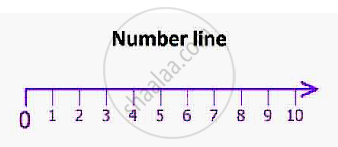HSC Arts 11thMaharashtra State Board
Share

# Subsets

#### description

• Subsets of a Set of Real Numbers Especially Intervals - With Notation
• Singleton Set
• Super Set
• Subsets of set of real numbers
• Intervals as subsets of R

#### definition

A 'set A' is said to be a subset of a set B if every element of A is also an element of B.

#### notes

Sub means 'part of', thus subset means part of set.
B= {1, 2, 3, 4, 5} and A= {1, 2}
A set is the part of set B
A is subset of B
Mathematically it is written as A ⊂ B
'⊂' means is subset of
B= {1, 2, 3, 4, 5}, A= {1, 2}, C= {3, 4}, D= {3, 5} and E= {1,5}
A, C, D and E are subsets of B
Mathematically, if A ⊂ B, a ∈ A ⇒ a ∈ B
'a' is an element of set A
'⇒' means implies
If A is not the subset of B, then mathematically we will write A ⊄ B
'⊄' means not the subset of
Case 1- If every elemento of A is also an element of B, i.e A ⊂ B
But if every element of B is also an element of A, i.e B ⊂ A
then A=B
A ⊂ B and B ⊂ A ⇔ A= B
'⇔' means two way implication
Note: 1) A = A
A⊂ A. Every set is subset of itself.
2) Ø  ⊂ any set . Empty set is subset of any set.

There are many important subsets of R (set of all real numbers). We give below the names of some of these subsets.

The set of natural numbers N = {1, 2, 3, 4, 5, . . .}
The set of integers Z = {. . ., –3, –2, –1, 0, 1, 2, 3, . . .}
The set of rational numbers Q = { x : x = p/q, p, q ∈  Z and q ≠ 0}
The set of irrational numbers, denoted by T, is composed of all other real numbers. Thus T = {x :  x ∈ R and x ∉ Q}, i.e., all real numbers that are not rational.
Members of T include  2 , 5 and π .
Some of the obvious relations among these subsets are:
N  ⊂  Z ⊂ Q, Q ⊂ R, T ⊂ R, N ⊄ T.
Let A & B be two sets. A ⊂ B and A ≠ B.
Then A is called proper subset. and B is called superset.
A set containing only one element is known as Singleton set.

A= {1}, B= {Ø}, C= {2}

Intervals as subset of R
If A= {x: 5 ≤ x ≤ 9 ∀ x ∈ R}Real Interval [5,9] is subset of R, here 5 and 9 are included therefore we wrote them in these '[ ]' brackets and such intervals are known as closed intervals.
If B= {x:5 < x  < 9, ∀ x ∈ R}
Real Interal (5,9) is subset of R, here 5 and 9 are excluded therefore we wrote them in these '( )' brakets and such intervals are known as open intervals.
If C= {x: 5 < x ≤ 9 ∀ x ∈ R}
Real interval (5,9] is subset of R, here 5 is excluded and 9 is included therefore we wrote them in '(' and ']' brackets such intervals are known as open closed intervals.
If  D= {x: 5 ≤ x < 9, ∀ x ∈ R}
Real interval [5,9) is subset of R, here 5 is included and 9 is excluded therefore we wrote them in '[' and ')' brackets such intervals are known as closed open intervals.

Length of Interval: if [a,b], (a,b), [a,b), (a,b] are the subsets, then b-a is the length of interval.

### Shaalaa.com

Subset [00:02:38]
S
0%

S